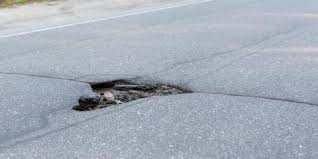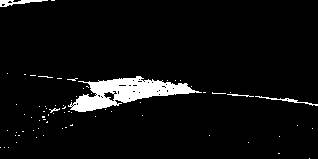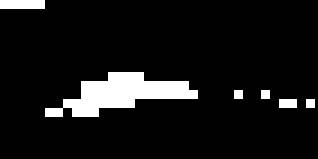The purpose of this project is to build an algorithm for detecting whether there is a pothole on a road from the image of the road. The work-flow is as follows:

1. Build a set of road images
• 15 images of roads with potholes
• 10 images of roads with no potholes
1. Extract pothole segments from images
• Segment pothole like sections of the image using Histogram shape-based threshold
• Mark the sections that look like potholes
1. For each image, compute the features that determine whether there is a pothole.

2. Classify the image based on a decision rule.

We use the algorithms mentioned in the following reference:

Reference : Koch, C, Brilakis, I Pothole detection in asphalt pavement images

## Bulding The Image Set

Each image is in color and with .jpg extension. When we load them using jpeg package, they are loaded as arrays. The first two dimensions represent the row and columns of the pixed. The third dimesion contains the RGB values. The images are converted to grey by taking the average of RGB values for each pixel.

## Image Segmentation

The following algorithm is used segment the pothole like sections:

1. Convert the grey values of each pixel to 0-255 sclae. Build a histogram of grey values.

2. Find the maximum point on the histogram graph (the grey value that occurs most frequently). Let’s call this point (G, h(G)), where G denotes the most frequent grey value.

3. Determine a threshold T is as the intensity value of a histogram point (T, h(T)), which has the maximum distance to the line that joins the origin (0, 0) of the histogram to the point (G, h(G)).

4. Mark all the points which have a grey value lower than threshold T as pothole candidates.

SegmentPothole <- function(img){
dim_pothole <- dim(img)

pothole_grey <- matrix(data=0, nrow = dim_pothole,
ncol=dim_pothole)

sapply(1:dim_pothole, function(i){
sapply(1:dim_pothole, function(j){
pothole_grey[i,j] <<- mean(img[i,j,])
})
})

pothole_vec <- round(as.double(pothole_grey)*255)

val_count <- as.data.frame(table(pothole_vec))
setDT(val_count)
val_count$pothole_vec <- as.numeric(as.character(val_count$pothole_vec))

hist_maxima <- unlist(matrix(val_count[which.max(Freq)])[,1])

val_count[, perp_dist := abs(hist_maxima * pothole_vec -
hist_maxima * Freq)/sqrt(sum(hist_maxima^2))]

grey_thresh <- val_count[pothole_vec < hist_maxima][which.max(perp_dist)]\$pothole_vec/255

pothole_segmented <- array(data=0, dim = c(dim_pothole,
dim_pothole, 3))

pothole_segmented_mat <- matrix(data=0, nrow = dim_pothole,
ncol =dim_pothole)

sapply(1:dim_pothole, function(i){
sapply(1:dim_pothole, function(j){
if(mean(img[i,j,]) <= grey_thresh){
pothole_segmented[i,j,] <<- 1
pothole_segmented_mat[i, j] <<- 1
}
})
})

return(list(pothole_grey, pothole_segmented, pothole_segmented_mat))

}

Shown below are the results of the above code on a particular input image file.input imageoutput segmented image

## Mark Pothole Segments

The following heuristic is used to mark the pothole segments:

1. Split the image into 9X9 non-overlapping boxes.

2. If more than 15% of the pixels in the box are marked as pothole candidates, mark the whole box as pothole candidate.

ExtractPotholeRegions <- function(img){

dim_pothole <- dim(img)

segment_list <- SegmentPothole(img)

pothole_grey <- segment_list[]
pothole_segmented <- segment_list[]
pothole_segmented_mat <- segment_list[]

row_seq <- seq(1, dim_pothole, 9)
col_seq <- seq(1, dim_pothole, 9)

pothole_mat <- matrix(data=0, nrow = dim_pothole,
ncol=dim_pothole)

pothole_sec <- array(data=0, dim = c(dim_pothole,
dim_pothole, 3))

sapply(1:(length(row_seq)-1), function(i){
sapply(1:(length(col_seq)-1), function(j){
curr_section <- as.double(pothole_segmented_mat[row_seq[i]:min(row_seq[i+1]-1, dim_pothole),
col_seq[j]:min(col_seq[j+1]-1, dim_pothole)])

if(sum(curr_section)/length(curr_section) > 0.15){
pothole_mat[row_seq[i]:min(row_seq[i+1]-1, dim_pothole),
col_seq[j]:min(col_seq[j+1]-1, dim_pothole)] <<- 1
pothole_sec[row_seq[i]:min(row_seq[i+1]-1, dim_pothole),
col_seq[j]:min(col_seq[j+1]-1, dim_pothole), ] <<- 1
}

})
})

return(list(pothole_grey, pothole_segmented, pothole_mat, pothole_sec))

}

The result of the above code is shown below:output Marked image

## Features

Given below is a 9X9 Laplacian of Gaussian filter (LOG).

##  "Laplacian Gaussian Filter"
##       [,1] [,2] [,3] [,4] [,5] [,6] [,7] [,8] [,9]
##  [1,]    0    0    3    2    2    2    3    0    0
##  [2,]    0    2    3    5    5    5    3    2    0
##  [3,]    3    3    5    3    0    3    5    3    3
##  [4,]    2    5    3  -12  -23  -12    3    5    2
##  [5,]    2    5    0  -23  -40  -23    0    5    2
##  [6,]    2    5    3  -12  -23  -12    3    5    2
##  [7,]    3    3    5    3    0    3    5    3    3
##  [8,]    0    2    3    5    5    5    3    2    0
##  [9,]    0    0    3    2    2    2    3    0    0

The following steps are performed for building features:

1. Perform convolution of the grey values of the image with a 9X9 LOG.

2. Compute standard deviation of the results of LOG filter for pothole segments (sd_pothole_LOG).

3. Compute standard deviation of the results of LOG filter for no pothole segments (sd_nopothole_LOG).

4. Compute standard deviation of the grey values of pothole segments (sd_pothole_grey).

5. Compute standard deviation of the grey values of no pothole segments (sd_nopothole_grey).

6. Feature 1 : sd_diff_grey = sd_pothole_grey - sd_nopothole_grey

7. Feature 2 : sd_diff_LOG = sd_pothole_LOG - sd_nopothole_LOG

## Pothole Prediction

If sd_diff_grey > 0 and sd_diff_LOG > 0, the image is calssified as Pothole, otherwise as No Pothole.

options(warn=-1)
library(jpeg)
library(data.table)

GetPotholeFeatures <- function(img){

dim_pothole <- dim(img)

extract_list <- ExtractPotholeRegions(img)

LOG_filter <- matrix(data= c(0,0,3,2,2,2,3,0,0,
0,2,3,5,5,5,3,2,0,
3,3,5,3,0,3,5,3,3,
2,5,3,-12,-23,-12,3,5,2,
2,5,0,-23,-40,-23,0,5,2,
2,5,3,-12,-23,-12,3,5,2,
3,3,5,3,0,3,5,3,3,
0,2,3,5,5,5,3,2,0,
0,0,3,2,2,2,3,0,0), nrow=9, ncol=9)

pothole_LOG <- matrix(data=0, nrow = dim_pothole,
ncol=dim_pothole)

pothole_grey <- extract_list[]
pothole_mat <- extract_list[]

sapply(1:(dim_pothole-8), function(i){
sapply(1:(dim_pothole-8), function(j){
curr_LOG <- pothole_grey[i:(i+8), j:(j+8)] %*% LOG_filter

pothole_LOG[i+4, j+4] <<- curr_LOG[5,5]
})
})

pothole_count <- sum(pothole_mat)
pothole_grey_vals <- matrix(data=0, nrow = pothole_count, ncol=1)
nopothole_grey_vals <- matrix(data=0, nrow = dim_pothole * dim_pothole -pothole_count, ncol=1)
pothole_LOG_vals <- matrix(data=0, nrow = pothole_count, ncol=1)
nopothole_LOG_vals <- matrix(data=0, nrow = dim_pothole * dim_pothole -pothole_count, ncol=1)

curr_ph_index <- 1
curr_noph_index <- 1

sapply(1:dim_pothole, function(i){
sapply(1:dim_pothole, function(j){
if(pothole_mat[i,j] == 1){
pothole_grey_vals[curr_ph_index, 1] <<- pothole_grey[i, j]
pothole_LOG_vals[curr_ph_index, 1] <<- pothole_LOG[i, j]
curr_ph_index <<- curr_ph_index+1
}else{
nopothole_grey_vals[curr_noph_index, 1] <<- pothole_grey[i, j]
nopothole_LOG_vals[curr_noph_index, 1] <<- pothole_LOG[i, j]
curr_noph_index <<- curr_noph_index+1
}

})
})

sd_grey <- sd(pothole_grey_vals[, 1]) - sd(nopothole_grey_vals[, 1])
sd_LOG <- sd(pothole_LOG_vals[, 1]) - sd(nopothole_LOG_vals[, 1])

return(list(extract_list[], extract_list[], sd_grey, sd_LOG))

}

GetPotHoleFreaturesAll <- function(input_path){

file_names <- list.files(input_path, patter='*.jpg')

results <- NULL

lapply(file_names, function(file_name){

print(file_name)

curr_features <- GetPotholeFeatures(curr_image)

curr_table <- data.table(file_name = file_name,
sd_grey = curr_features[],
sg_LOG = curr_features[],
pred = ifelse(curr_features[] > 0 &&
curr_features[] > 0,
'pothole',
'nopothole'))

results <<- rbind.data.frame(results, curr_table)
})

return(results)

}

pothole_results <- GetPotHoleFreaturesAll('/Users/apple/Desktop/PotholeDetection/pothole_images/')

nopothole_results <- GetPotHoleFreaturesAll('/Users/apple/Desktop/PotholeDetection/nopothole_images/')

## Results

The overall accuracy of pothole prediction on 25 test images is 80%. The accuraccy was similar on both the samples containing potholes and the samples contaning no potholes.

Prediction On Pothole Images
pred N
pothole 12
nopothole 3
Prediction On No Pothole Images
pred N
nopothole 8
pothole 2

## Conclusion

This simple algorithm can be used to detect potholes on the raods from their images. It can have applications in road repair operations.